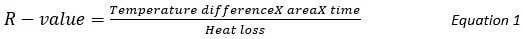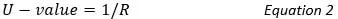# Details about R-value and U-value of Concrete SlabThe conduction of heat regarding the materials utilized in its construction is measured with the thermal conductivity coefficient alias k-value (W/m.K), of the materials used in its construction. It belongs to the rate at which heat moves through a material among points at dissimilar temperatures.

The thermal resistance alias R-value (m2K/W), is computed by dividing the thickness of the material (in metres) with the k-value. From this the thermal transmittance alias U-value (W/m2.K) concerning a building material is measured like the inverse of the sum of the R-values of the component parts and adjoining air layers.

R-value stands for the estimation of concrete slab (or other material) strength to counter heat flow i.e. it calculates the potency of insulation or thermal resistance. However, U-value stands for the estimation of heat transmission throughout concrete slab from ground into the closed space or oppositely.

Since, thermal insulation fluctuates contrariwise with density, lower density concrete offers superior insulation as compared to greater density concrete. With the purpose of determining the strength of the reinforced concrete slab to withstand heat transfer, it is necessary to estimate R-value and U-value of the reinforced concrete slab under consideration.

The method for estimating R-value for Concrete Slab: A perfect R-value for a normal concrete slab is computed with an R-value, the thermal resistance per inch of thickness, among 0.1 and 0.2 and multiplying it times the slab thickness. The value of R is determined with the following equation provided by ASTM C 168:Here, the temperature variation (among exterior and interior of concrete slab) is provided in degrees Fahrenheit, the area is given in square feet, the time in hours, and the heat loss in Btus.

ASTM C 168 also offers two supplementary expressions to measure R-value and is available in ASTM C 168 document.

The R-value imperial unit and metric unit are given below:

Computation of u-value for Concrete Slab

The U-value for a concrete slab (and any other building material) stands for the inverse of its R-value and is computed with the formula given below:The U-value unit is the inverse those of R-value:Note: There is difference among the American Standard and European Standard for R-value and U-value. Therefore, to transform an American R-value into a European U-value, divide 1 with the R-value, then multiply the result by 5.682 whereas transforming a European U-value to an American R-value, multiply with 0.176, then divide 1 by the result.